Puzzle Page 2 *************************************** Puzzle #47       12/1/2002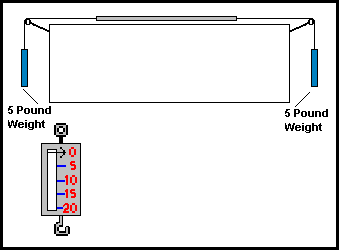Pictured above on the left is a scale for weighing small objects up to 20 pounds. It is meant to be held by the top ring and the object to be weighed goes on the hook. However, the weight of the scale is so light it works the same upside down. For example, if you were to hold it by the ring and place a 20 pound object on the hook OR if you held it upside down by the hook and clipped the object on the ring it would indicate 20 pounds in both cases. On the top of the diagram, the scale has been placed horizontally on a table with ropes affixed to the ring on one side and the hook on the other side. The ropes travel over pulleys which are attached to five pound weights on each side. Assuming the ropes have negligible weight and the pulleys have negligible friction, the question is what will the scale read? You could reason that the 5 pound weight on the left cancels the five pound weight on the right and it reads zero. OR you could reason that with 5 pounds on the left and 5 pounds on the right, it must be 5 pounds in the middle too. OR you could say that since there are 5 pounds on the left and right then the scale must read 10 pounds. OR is the answer something else entirely? ANSWER The tension in the rope is the same throughout its entire length. Although it seems counter-intuitive, the scale will read 5 pounds !! Remember, in this example we are NOT measuring mass but weight. *************************************** Puzzle of the Month Is "On Vacation" *************************************** Puzzle #46       5/1/2002 What do these 5 words have in common? astute   undefined   monopoly   hijinks   thirsty ANSWER All of the words contain 3 letters in alphabetical order: aSTUte   unDEFined   moNOPoly   HIJinks   thiRSTy *************************************** Puzzle #45       4/1/2002 This is not an April Fool's prank. Your job is to find out why this ordinary-looking paragraph is so unusual. At first, it looks fairly common and unimportant. It's just a plain insignificant paragraph right? No - not at all. Don't think that this has a 'trick' solution, but don't think you will find an instant solution. No luck on your first try? Try again. In fact, you could look at this for a third, fourth or fifth try and probably still not hit upon what it is that puts this paragraph in a class all its own (or almost). Only a small handful of paragraphs (if that) can lay claim to this paragraph's particular curious trait. You could look at this again and again and still not know how unusual it is. ANSWER Following that first paragraph's unusual quality, this solution too will carry on (up to a point) without utilizing a common trait that is found in a vast majority of writing. And just what is this limitation? What is prohibiting both paragraphs from having just an ordinary status? If you could not find a solution by now, you should quit and go on to that third paragraph. Once again, Joe Cool (a frequent reader of this Puzzle Page) figured out the answer. The letter 'E' is not used in either of those paragraphs !! It is the most common letter in the English language ! It is so common that the letter 'E' was used 14 times just in the previous 2 sentences ! Yes it is quite difficult to write anything intelligible for any length of time without using an 'E'. The letter 'E' appears in very common words - 'the' 'there' 'he' 'she' 'we' and so on. How about trying to express anything in the past tense? Just about everything in the past tense ends in 'ed'. As an interesting bit of trivia, the American author, Ernest Vincent Wright, wrote a novel entitled "Gadsby". In that entire novel of about 50,000 words, the letter 'E' does not appear at all! If you wish to read that book, someone has posted it online Gadsby - the book withour an 'e'. *************************************** Puzzle #44       3/1/2002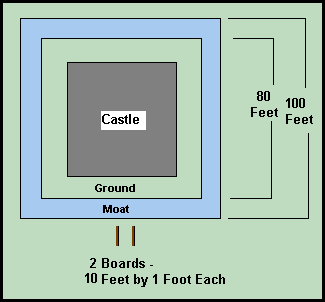You are back in medieval times and you have the following predicament. You have to reach the castle but that 10 foot wide moat prevents you from doing so. The only items available to you are two 10 foot boards. Even though each board is just barely too short, you still can use these to cross the moat safely so you can rescue the kidnapped princess, claim your rightful heritage to the throne, retrieve the Holy Grail, stop the sheriff from harming Maid Marion, or do some other thing that they did in Medieval times. How do you use the boards to cross the moat? ANSWER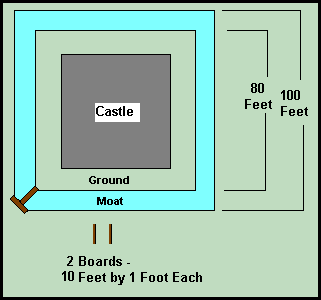Once again "Joe Cool" (a fan of this Puzzle Page) figured out the answer !!! *************************************** Puzzle #43       2/1/2002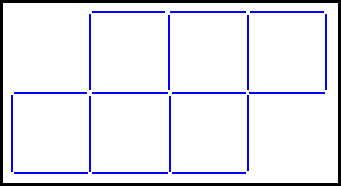In the above diagram, 6 squares are made from 18 toothpicks. Just by moving (NOT removing) 2 toothpicks, make 5 equally sized squares. ANSWER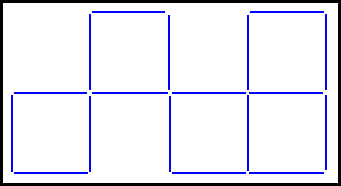*************************************** Puzzle #42       1/1/2002 What would be the product (or result) if we multiplied the 26 items in this algebraic expression ? (x-a) * (x-b) * (x-c) * ....... * (x-z) ANSWER We could start out by multiplying the first 2 terms and obtaining: x2 -ax - bx +ab Then we could continue and multiply this by (x-c) but it would get very cumbersome very quickly. Instead, if we think about it, is there anything special about any of the terms? Well, consider the 24th term (x-x) which equals zero. Whenever any multiplication has a zero as a factor then the answer is always ZERO. *************************************** Puzzle #41       12/1/2001 The number 12 has 4 divisors - 2, 3, 4, and 6. The number 15 has only 2 divisors - 3 and 5. What 3 digit number has the MOST divisors ? ANSWER The number 840 has 30 divisors: 2, 3, 4, 5, 6, 7, 8, 10, 12, 14, 15, 20, 21, 24, 28, 30, 35, 40, 42, 56, 60, 70, 84, 105, 120, 140, 168, 210, 280 and 420 *************************************** Puzzle #40       11/1/2001 What English word has all the vowels in alphabetical order ? ANSWER The word is "facetiously". (Another answer, though not a very commonly used word, is "abstemiously"). *************************************** Puzzle #39       10/1/2001 What 6 letter English word contains only 1 vowel - the letter "y" ? ANSWER The word is "rhythm". Another answer is the word "nymphs" (again, submitted by "Joe Cool"). Also, the word "rhythms" is a SEVEN letter word with the only vowel being the letter "y". *************************************** Puzzle #38       9/1/2001 What word in the English language contains 5 consonants in a row? Another word, not quite as common contains 6 consonants in a row. ANSWER The words are "witchcraft" and "latchstring". "Joe Cool" (a fan of this page) suggested the word "nightstick" as another word that has 5 consonants in a row. *************************************** Puzzle #37       8/1/2001 Okay, another word puzzle. What word in the English language contains no vowels? (doesn't even contain 'y' either.) Oh, and this is not one of those stupid trick questions such as "What's the longest word? 'Smiles' because it has a mile between each 's'." Don't you hate those? Anyway, this 'no vowel word' is not some obscure word for 'Medieval axe handle sheath'. It is not too common but it is a word I'm sure we've all heard. It can be found in any English dictionary. Are you totally confused? Okay, enough talk. See you in a month. ANSWER The word is "nth". Look it up. It's in any English dictionary. *************************************** Puzzle #36       7/1/2001 Okay, it's word problem time once again. What 9 letter word has only 1 syllable and only 1 vowel ? ANSWER The word is STRENGTHS. *************************************** Puzzle #35       6/1/2001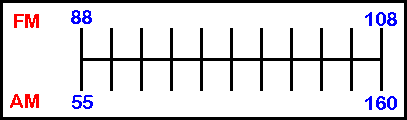Back in the "old days" before digital tuning, radios were tuned by a dial which would move a pointer back and forth across a scale (see above). This scale was usually numbered in the way it is illustrated above. The FM scale is in MegaHertz and the AM scale is in KiloHertz divided by ten. At what point in this diagram are the AM and FM numbers equal ? ANSWER First we must determine the rate at which both scales change. 160 - 55 = 105 and 108 - 88 = 20 105/20 = 5.25 . Therefore, for every "FM Increase", AM increases 5.25. Now, we must figure out some conversion formulas. 1)   FM = (AM + 407) / 5.25 and 2)   AM = (FM * 5.25) -407 Since, we are looking for equal AM & FM numbers, we will set equation1 equal to equation 2. (AM + 407) / 5.25 = (FM * 5.25) -407 This reduces to: AM = 27.5625 * FM -2,543.75 Substituting equation 2 into the left side of the equation we obtain: (FM * 5.25) -407   =   27.5625 * FM -2,543.75 This reduces to: FM = 95.76470588....... and therefore, AM = 95.76470588....... Bet you didn't think it would be that difficult did you? *************************************** Puzzle #34       5/1/2001 How about another word problem? This one involves rearranging the letters of words into alphabetical order. For example the word 'table' arranged alphabetically is 'abelt'. The word 'window' becomes 'dinoww' and so on. Arranging words in this way and then ordering them alphabetically, what word would be last in this list? (Confine your search to common words). ANSWER As far as common words are concerned, the word "US" would be last on the list. Going to obscure words, "TUTU" is even further down the list. Still further down the list is the slang word for tuxedo --- "TUX". *************************************** Puzzle #33       4/2/2001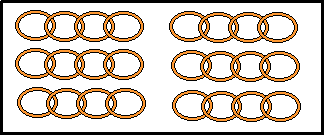For one thing, notice the date of this puzzle is April 2nd just in case you think this might be an April Fool's Day "trick puzzle". Okay. Referring to the above diagram, there are 6 chains consisting of 4 links each. You want to make one long 24 link chain so you go to the local welder who says "it costs 2 dollars to open and reweld a link so that will be 10 bucks." There is a way to do this for less than 10 dollars. How? ANSWERIf you open the last link of chain #1 and connect it to the first link of chain #2, and continue to do this up to chain #6 it does cost \$10.00 However, if ALL the links of chain #1 are opened up and separated, one link can be used to connect chain #2 to chain #3, the next link to connect chain#3 to chain #4 and so on, making the cost only \$8.00. *************************************** Puzzle #32       3/1/2001 How about a word puzzle for a change? There are 4 common English words that end in 'dous'. Three of these words rhyme with each other, making the fourth word the most difficult to think of. What are these four words? ANSWERThe words are "STUPENDOUS", "TREMENDOUS", "HORRENDOUS" and "HAZARDOUS". *************************************** Puzzle #31       2/1/2001 What is the next number in this series? 1     2     5     14     42     132   429     ? ANSWERThese are known as "Catalan Numbers" and they are generated by multiplying the previous Catalan Number by (4n-2)/(n+1). For example, the sixth Catalan Number is 132. So, to derive the seventh, multiply 132 times (4*7-2)/(7+1) and this equals 429. So, the eighth Catalan Number equals 429 times (4*8-2)/(8+1) which equals 1,430. Catalan Numbers have some interesting properties and uses. Again, use your favorite Search Engine to find out more about them. *************************************** Puzzle #30       1/1/2001 We have discussed factorial numbers before (see Puzzle 26 for example). The number 20 factorial is equal to 2,432,902,00?,176,640,000. Notice that we have replaced one of the digits with a question mark. It is possible to determine this digit without doing any multiplication. What is the missing digit? ANSWER The digits of multiples of 9 always add up to nine. For example,let's sum the digits of 387 (which is 9 x 43). 3 + 8 + 7 = 18 and 1 + 8 = 9. (This is called a 'digital root' by the way). Six factorial is a multiple of 9, therefore, any factorial greater than this will be a multiple of 9. So, 2 + 4 + 3 + 2 + 9 + 0 + 2 + 0 + 0 + ? + 1 + 7 + 6 + 6 + 4 = 46 4 + 6 =10 and therefore the missing digit is an EIGHT. *************************************** Puzzle #29       12/1/2000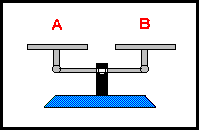Weighing objects with a double-pan balance (see above) is done by placing an object on one pan and placing weights on the other pan until it equals the weight of the object on the other pan. Usually, the weights are based on powers of 2. For example, with ten weights of 1, 2, 4, 8, 16, 32, 64, 128, 256 and 512 grams any object of 1 to 1,023 grams can be weighed. For example, a 5 gram object on pan A will be balanced off by weights of 4 & 1 gram on the other. In some cases, it is easier to put weights on both pans. For example, a 255 gram object on pan A could be balanced off with weights of 1, 2, 4, 8, 16, 32, 64 and 128 grams OR with the 1 gram weight on pan A and the 256 gram weight on pan B. (256 grams minus 1 gram = 255 grams). Actually, you could make ALL weighings from 1 to 1,023 grams using only 7 weights. What are the values of these seven weights? ANSWER The weights would be in powers of 3 - that is 1, 3, 9, 27, 81, 243 and 729. By using both pans, any amount up to 1,093 grams can be weighed. For example, a 5 gram object can be weighed with that object and the 3 and 1 gram weights on one pan and the 9 gram weight on the other. *************************************** Puzzle #28       11/1/2000 In the diagram below, 2 quarters are represented by circles A & B. If you hold quarter B stationary and rotate quarter A around B you will find that it will undergo 2 rotations. (Intuitively, you'd think it would just be one wouldn't you?). Now suppose that circle A is 1 inch in diameter and that circle B is 10 inches in diameter, how many rotations would circle A undergo?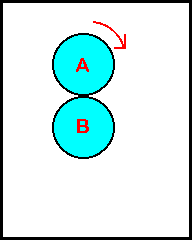ANSWER The trick to this problem is that the coin (or circle) will always make one extra rotation no matter how big or small the other circle is. For example, if you were to get a pin (which has almost NO diameter), you will find that a coin will still make one rotation around it. So for the 1 inch circle around the 10 inch circle, the answer would be ELEVEN rotations. *************************************** Puzzle #27       10/1/2000 I call this puzzle "Splitting The Number Line". Writing consecutive integers starting from 1, will there ever be a case where the sum of 1 to 'n' equals the sum of (n+1) to a number we shall call 'x'? Well, the first such occurrence is 1 + 2 = 3. The next occurrence is 1 through 20. That is the sum of 1 through 14 equals the sum of 15 through 20 which equals 105. What is the next such occurrence? ANSWER A handy formula for adding consecutive integers is: n*(n+1)/2. For example, the sum of the integers 1 through 100 equals: 100*(101)/2     = 5,050 By using this formula and a 'trial and error' search. the next such instance is: 1 through 84 = 85 through 119 = 28,561 If you are curious, the next 8 instances are: 1 through 492 = 493 through 696 = 121,278 1 through 2,870 = 2,871 through 4,059 = 4,119,885 1 through 16,730 = 16,731 through 23,660 = 139,954,815 1 through 97,512 = 97,513 through 137,903 = 4,754,343,828 1 through 568,344 = 568,345 through 803,760 = 161,507,735,340 1 through 3,312,554 = 3,312,555 through 4,684,659 = 5,486,508,657,735 1 through 19,306,982 = 19,306,983 through 27,304,196 = 186,379,786,627,653 1 through 112,529,340 = 112,529,341 through 159,140,519 = 6,331,426,236,682,470 Notice that each instance is larger than the previous instance by a factor of 5.828.... the limit of which is 3 plus the square root of 8. *************************************** Puzzle #26       9/1/2000 Fifteen employees decide to buy holiday presents for each other by the following procedure. Each of the fifteen names is written on a piece of paper, placed in a bowl and then each employee selects 1 name. The only rule is that if you draw your own name, the whole process must be restarted. What is the probability that all 15 employees will NOT draw their own name on the first "round"? ANSWER There are 15 ! (meaning 15 factorial or 15x14x13x12x11x10x9x8x7x6x5x4x3x2x1) ways for the 15 employees to draw the names. (That's 1,307,674,368,000 ways, in case you were curious). Because this number is gigantic, let's do some calculations with the smaller numbers. If it were 2 employees, we can easily illustrate both of the 2 ! outcomes: 1)Employee 1 draws #1 ; Employee 2 draws #2 and 2)Employee 1 draws #2 ; Employee 2 draws #1. There is only 1 "success" for 2 employees so the probability is .5. For 3 employees, there are 3 ! (or 6) outcomes: EMPL #1     EMPL #2     EMPL #3 1                2                 3 1                3                 2 2                1                 3 2                3                 1 3                1                 2 3                2                 1 There are 2 "successes" (the fourth and fifth outcomes) and so for three employees, the probability is 2/6 or .33333333333... For 4 employees, there are 4 ! (or 24) outcomes. It gets a little awkward trying to illustrate these results on the computer, so you'll have to take our word, (or try it yourself with pen and paper) that there are 9 "successes". So, probability = 9/24 or .375 exactly. For 5 employees, there are 5 ! (or 120) outcomes. There are 44 "successes". So, probability = 44/120 or .36666666666666... For 6 employees, there are 6 ! (or 720) outcomes. There are 265 "successes". So, probability = 265/720 or .3680555555555... The clever among you may have noticed some patterns developing here. a) Rather quickly, the probability starts "hovering" around .36 as the number of employees (or 'n') increases. b) When 'n' is even, the probability is a little high, and it's a bit on the low side when 'n' is odd. c) This one's a little complicated but here goes. What is the precise amount of "successes" for each value of 'n'? By the "brute force" method of writing them, we see that for n=2 there is 1 success n=3     2 successes n=4     9 successes n=5   44 successes n=6 265 successes So, what is the pattern here? For each 'n', take the previous number of successes and multiply it by 'n'. If 'n' is even, add 1 to this product, if 'n' is odd, subtract 1. For example, for 'n' =4, successes = (2 x 4) + 1 which equals 9. For 'n' = 5, successes = (9 x 5) -1 equaling 44. For 'n' = 6, successes = (44 x 6) + 1 = 265. From this pattern, we can determine the values all the way to 15. n=7     1,854 n=8     14,833 n=9     133,496 n=10   1,334,961 n=11   14,684,570 n=12   176,214,841 n=13   2,290,792,932 n=14   32,071,101,049 n=15   481,066,515,734 Incidentally, these permutations that have no elements in their proper positions are called derangements. So, for those of you that want the precise answer (and for those of you who have managed to stay awake), the probability is (drum-roll please)... 481,066,515,734   divided   by   1,307,674,368,000   which   equals 0.367879441171397... As 'n' increases the probability approaches the value of 1 ÷ e. What is 'e'? Good question. It is an important mathematical constant (but probably doesn't have the same 'press agent' as "pi" does). Among other things, 'e' is the basis of natural logarithms. As usual, if you want more information about 'e' use your favorite search engine. Maybe Cliff's Notes™ could write a summary of this answer? *************************************** Puzzle #25       8/1/2000 You've seen "Magic Squares" where numbers are arranged in which every row, column and diagonal equals the same sum. There are many methods for constructing such squares. However, the 3 by 3 square can be constructed just by using logic and reasoning. So the problem is, arrange the numbers 1 through 9 in a 3 by 3 square such that each row column and diagonal totals 15. ANSWER Because each row, column and diagonal must sum to 15, there are certain numbers which cannot be paired with other numbers. Nine for example, can't be paired with eight (because 9 + 8 = 17) seven (because 9 + 7 = 16) six (because 9 + 6 = 15) three (because 9 + 3 = 12, which would require the use of another 3). By similar reasoning, we conclude that Seven cannot be paired with the numbers nine, eight and four. Three cannot be paired with the numbers one, two and six. One cannot be paired with the numbers two, three four and seven. This would prevent the numbers Nine, Seven, One and Three from being placed in certain boxes of the 3 by 3 grid. The center is eliminated because any of these numbers must be paired with ALL the other numbers. Similarly, nine, seven, three and one cannot be placed in any of the four corners because a corner number is only unshared with 2 other numbers. Therefore, nine, seven, three and one must be placed in the four "side" locations of the 3 by 3 grid. How should they be placed? For reasons stated above, the nine must be placed opposite the one and the seven must be placed opposite three. To get the sum to equal 15, the 5 must be placed in the center. Then, it is a simple matter to place the remaining four numbers and your answer will be: 8       1       6 3       5       7 4       9       2 If you want to learn more about "Magic Squares", try these links: Making Odd Magic Squares Making Doubly Even Magic Squares (Meaning Divisible by 4) Making Singly Even Magic Squares (Meaning Divisible by 2 but not by 4) *************************************** Puzzle #24       7/1/2000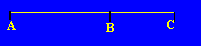In the above figure, the ratio of Line AB to Line BC is the same as the ratio of Line AC to Line AB. Basically, this is saying:   AB ÷ BC = AC ÷ AB What is this ratio? ANSWER We will say that the entire line AC will equal 1. Let BC = x and that means that AB will equal 1-x. According to the problem, the ratio of (1-x)/x equals 1/(1-x). This equation becomes: x2 -3x +1 =0 By the quadratic formula we obtain x= .381966011 This means 1/x equals .618033988 So, 1/(1-x) = 1.6180339... Incidentally, this number is called the Phi Ratio. You might find it interesting to find out more about it. (Use your favorite Internet Search Engine).
__________________________